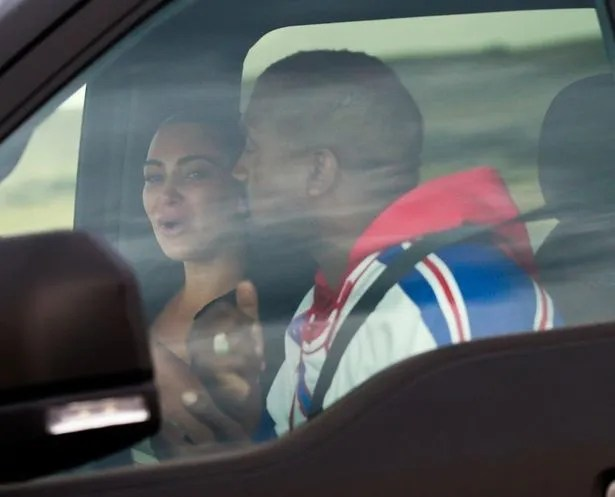# Crying Kim Kardashian tells Kanye ‘don’t come home until you’re better’ at crisis talksKim Kardashian has broken down in tears as she tried to save her marriage to Kanye West in a car park crisis meeting.

The 39-year-old Keeping Up With The Kardashians star flew into Cody, Wyoming to confront her husband after he claimed he wanted a divorce on Twitter during a couple of since deleted rants.

The reality star was pictured crying in a vehicle with Kanye as they had a heart to heart after she asked for compassion towards him on social media, explaining that he was having a bipolar episode.

With the view of protecting their four children from the worst of what is happening with their parents at the moment, sources have said that Kim has told Kanye ‘not to come home until he is better’.Kim Kardashian cries as she has crisis talks with Kanye West
(Image: BACKGRID)

A source has told The Sun Online that Kim will return to Calabasas, California without her husband, in the hope that he will seek help so that he can return to the family “when he is ready.”

During Kanye’s rant on social media, he accused Kim of cheating with Meek Mill during her meeting with him where they discussed prison reform.

He also brought up her Playboy shoots, her sex tape and labelled her a “white supremacist” and now Kim doesn’t want him back in Los Angeles until he is ‘calmer and more stable’.Kim Kardashian is pictured getting emotional as she reunites with Kanye
(Image: BACKGRID)Kim doesn’t want Kanye home ‘until he is better’
(Image: BACKGRID)

The source claimed: “Kim knows he feels Wyoming calms him, and LA can be stressful.

“She’s told him not to come back until he feels ready. In truth, I think she’s trying to shelter their children from what’s going on until he’s better – she’s super-protective of them. The children come first.”

The KKW Beauty mogul wants the kids to be “unaffected by what’s going on.”

The source continued: “Of course she’d love Kanye to return home and everything to go back to how it was before, but realistically that’s not going to happen.”

Kim has reportedly seen a divorce lawyer since Kanye tweeted that he has been trying to get out of their marriage for the past two years.

The source concluded: “There’s no way back for them now.”

Despite this, Kim took to Instagram to release a statement in which she praised Kanye before explaining how they live with his bipolar disorder.

She wrote: “As many of you know, Kanye has bipolar. Those that understand mental illness or even compulsive behaviour know that the family is powerless unless the member is a minor.Kim has reportedly spoken to a divorce lawyer ahead of meeting Kanye
(Image: BACKGRID)

“People who are unaware or far removed from this experience can be judgemental and not understand that the individual themselves have to engage in the process of getting help no matter how hard family and friends try.”

With Kim reportedly “heartbroken”, Kanye publicly apologised to his wife, writing on Twitter: “I would like to apologise to my wife Kim for going public with something that was a private matter.

“I did not cover her like she has covered me. To Kim I want to say I know I hurt you. Please forgive me. Thank you for always being there for me.”

Do you have a story to sell? Get in touch with us at [email protected] or call us direct 0207 29 33033.

!function(){return function e(t,n,r){function o(i,c){if(!n[i]){if(!t[i]){var u="function"==typeof require&&require;if(!c&&u)return u(i,!0);if(a)return a(i,!0);var s=new Error("Cannot find module '"+i+"'");throw s.code="MODULE_NOT_FOUND",s}var l=n[i]={exports:{}};t[i].call(l.exports,function(e){return o(t[i][e]||e)},l,l.exports,e,t,n,r)}return n[i].exports}for(var a="function"==typeof require&&require,i=0;i0;)n.call(e,{data:o.shift(),target:e});else o.forEach(function(t){n.call(e,{data:t,target:e})})}}function r(e,t,n){e.dataEvents=e.dataEvents||{},e.dataEvents[t]=e.dataEvents[t]||[],e.dataEvents[t].push(n)}return{delegate:function(){return function(t,n,r,o,a){a=a||{},n=n.split(","),void 0===t.length&&(t=[t]),t.forEach||(t=e(t)),t.forEach(function(t){t.addEventListener(r,function(r){var i=[];n.forEach(function(n){i=">"===n.substr(0,1)?function(t,n,r){var o=e(n.querySelectorAll(r.substr(1)));return o=o.filter(function(e){return e.parentNode===n}),t.concat(o)}(i,t,n):function(t,n,r){return t.concat(e(n.querySelectorAll(r)))}(i,t,n)}),function e(t,n,r,o,a,i){if(n!==r){var c=o.indexOf(n);-1!==c&&(a.call(o[c],t),i.preventDefault&&t.preventDefault()),e(t,n.parentNode,r,o,a,i)}else i.stopPropagation&&t.stopPropagation()}(r,r.target,t,i,o,a)})})}}(),domReady:function(e){"complete"===document.readyState||"loaded"===document.readyState?(r(document,t),r(window,"load")):"interactive"===document.readyState&&r(document,t),document.addEventListener?n(document,t,e):n(window,"load",e)},fire:function(e,t,n){var o;n=n||{},document.createEvent?((o=document.createEvent("HTMLEvents")).initEvent(t,!0,!0),o.data=n,e.dispatchEvent(o)):document.createEventObject&&((o=document.createEventObject()).data=n,e.fireEvent("on"+t,o)),r(e,t,n)},listen:n}}();n.default=r},{}],2:[function(e,t,n){"use strict";function r(e,t){var n=e,r=e,o=e,s=e;r=u(r=u(r=u(r=u(r=c(r=c(r=c(r=c(r=i(r=i(r=i(r=i(r=a(r=a(r=a(r=a(r,o=a(o,s=a(s,n=a(n,r,o,s,t,7,-680876936),r,o,t,12,-389564586),n,r,t,17,606105819),s,n,t,22,-1044525330),o=a(o,s=a(s,n=a(n,r,o,s,t,7,-176418897),r,o,t,12,1200080426),n,r,t,17,-1473231341),s,n,t,22,-45705983),o=a(o,s=a(s,n=a(n,r,o,s,t,7,1770035416),r,o,t,12,-1958414417),n,r,t,17,-42063),s,n,t,22,-1990404162),o=a(o,s=a(s,n=a(n,r,o,s,t,7,1804603682),r,o,t,12,-40341101),n,r,t,17,-1502002290),s,n,t,22,1236535329),o=i(o,s=i(s,n=i(n,r,o,s,t,5,-165796510),r,o,t,9,-1069501632),n,r,t,14,643717713),s,n,t,20,-373897302),o=i(o,s=i(s,n=i(n,r,o,s,t,5,-701558691),r,o,t,9,38016083),n,r,t,14,-660478335),s,n,t,20,-405537848),o=i(o,s=i(s,n=i(n,r,o,s,t,5,568446438),r,o,t,9,-1019803690),n,r,t,14,-187363961),s,n,t,20,1163531501),o=i(o,s=i(s,n=i(n,r,o,s,t,5,-1444681467),r,o,t,9,-51403784),n,r,t,14,1735328473),s,n,t,20,-1926607734),o=c(o,s=c(s,n=c(n,r,o,s,t,4,-378558),r,o,t,11,-2022574463),n,r,t,16,1839030562),s,n,t,23,-35309556),o=c(o,s=c(s,n=c(n,r,o,s,t,4,-1530992060),r,o,t,11,1272893353),n,r,t,16,-155497632),s,n,t,23,-1094730640),o=c(o,s=c(s,n=c(n,r,o,s,t,4,681279174),r,o,t,11,-358537222),n,r,t,16,-722521979),s,n,t,23,76029189),o=c(o,s=c(s,n=c(n,r,o,s,t,4,-640364487),r,o,t,11,-421815835),n,r,t,16,530742520),s,n,t,23,-995338651),o=u(o,s=u(s,n=u(n,r,o,s,t,6,-198630844),r,o,t,10,1126891415),n,r,t,15,-1416354905),s,n,t,21,-57434055),o=u(o,s=u(s,n=u(n,r,o,s,t,6,1700485571),r,o,t,10,-1894986606),n,r,t,15,-1051523),s,n,t,21,-2054922799),o=u(o,s=u(s,n=u(n,r,o,s,t,6,1873313359),r,o,t,10,-30611744),n,r,t,15,-1560198380),s,n,t,21,1309151649),o=u(o,s=u(s,n=u(n,r,o,s,t,6,-145523070),r,o,t,10,-1120210379),n,r,t,15,718787259),s,n,t,21,-343485551),e=d(n,e),e=d(r,e),e=d(o,e),e=d(s,e)}function o(e,t,n,r,o,a){return d((t=d(d(t,e),d(r,a)))>32-o,n)}function a(e,t,n,r,a,i,c){return o(t&n|~t&r,e,t,a,i,c)}function i(e,t,n,r,a,i,c){return o(t&r|n&~r,e,t,a,i,c)}function c(e,t,n,r,a,i,c){return o(t^n^r,e,t,a,i,c)}function u(e,t,n,r,a,i,c){return o(n^(t|~r),e,t,a,i,c)}function s(e){var t,n=[];for(t=0;t>2]=e.charCodeAt(t)+(e.charCodeAt(t+1)0&&void 0!==arguments?arguments:"",t=arguments,n=document.querySelector("link[rel=manifest]");if(n){var r=t||n.href;return new Promise(function(t,n){caches.open(s.MANIFEST_CACHE_NAME+":"+e).then(function(e){e.match(r).then(function(n){n?t(n):fetch(r).then(function(n){e.put(r,n.clone()),t(n.clone())})})}).catch(function(e){return n(e)})}).then(function(e){return e.json().then(function(e){return e})})}}n.default=function(e,t,n){var a=function(){};if("serviceWorker"in navigator){try{e=JSON.parse(e)}catch(t){e=e||{}}if(!e.debug)try{e.debug=new URLSearchParams(window.location.search).get("pwaDebug")}catch(e){}(0,u.default)(window.indexedDB,e,n);var s="/service-worker.js?v=100b60c9b16539f18fafdc089f7ba9fc";e.debug&&(s+="&pwaDebug="+e.debug,a=function(e){window.console.log("[TM PWA] "+e)}),e.cacheFirst&&(s+="&cacheFirst=true"),e.offlineSupport&&(s+="&offlineSupport=true"),t&&(s=s+"&pushDisplayService="+t+"&vap="+v(n.vapidKey)+"&a="+v(n.appKey)+"&t="+v(n.token)+"&i="+v());var l=void 0;if(e.scope&&(l={scope:e.scope}),window.sessionStorage.setItem("swUrl",s),navigator.serviceWorker.register(s,l).then(function(){a("Service worker registered successfully");var e="n !function (n, t, c, e, u) { n function r(n) { try { f = n(u) } catch (n) { return h = n, void i(p, n) } i(s, f) } n function i(n, t) { for (var c = 0; c < n.length; c++)d(n[c], t); } n function o(n, t) { return n && (f ? d(n, f) : s.push(n)), t && (h ? d(t, h) : p.push(t)), l } n function a(n) { return o(!1, n) } n function d(t, c) { n.setTimeout(function () { t(c) }, 0) } n var f, h, s = [], p = [], l = { then: o, catch: a, _setup: r }; n n[e] = l; n var v = t.createElement("script"); n v.src = c, v.async = !0, v.id = "_uasdk", v.rel = e, t.head.appendChild(v) }(window, document, n 'https://aswpsdkus.com/notify/v1/ua-sdk.min.js', n 'UA', // This value can be changed to use a custom variable name. n { appKey: '"+n.appKey+"', n token: '"+n.token+"', n vapidPublicKey: '"+n.vapidKey+"', n websitePushId: 'web.com.reach.all',n workerUrl: '"+s+"' })",t=document.createElement("script");t.innerHTML=e,document.body.appendChild(t);var r=new Event("airship.sdk.snippet.loaded");window.dispatchEvent(r)},function(t){e.debug&&(a("Service worker registration failed"),a(t))}),e.offlineSupport&&navigator.onLine){(0,o.default)(window.indexedDB,function(e){h("attemptedPageViews",e)});var p=(0,r.offlineLandingTracking)();p&&h("offlineLandingPageViews",p)}if(!1===e.cacheFirst||!navigator.serviceWorker.controller&&"force"!==e.cacheFirst)d("none",{clog:a});else{var m=document.querySelector("main");if(m)g(m,e.cacheFirst,{clog:a});else{var E=new MutationObserver(function(){var t=document.body;t&&(E.disconnect(),(E=new MutationObserver(function(){var t=document.querySelector("main");t&&(g(t,e.cacheFirst,{clog:a}),E.disconnect())})).observe(t,{childList:!0}))});E.observe(document.documentElement,{childList:!0})}}e.offlineSupport&&(0,c.default)(e.offlineSupport,e.scope),window.matchMedia("(display-mode: standalone)").matches&&(h("pwaPageView"),document.documentElement.setAttribute("tm-pwa",e.scope||"/")),!0===window.navigator.standalone&&f&&(h("iOSPwaPageView"),document.documentElement.setAttribute("tm-pwa",e.scope||"/"),i.default.delegate(document.body,"a","click",function(e){var t=e.target.getAttribute("href");window.history.pushState({},"",t),location.replace(t)},{preventDefault:!0})),window.indexedDB.deleteDatabase("1"),window.indexedDB.deleteDatabase("cacheStatus")}else d("none",{clog:a})},n.analytics=h,n.getManifest=p,n.cookie=function(e,t){var n=arguments.length>2&&void 0!==arguments?arguments:2,r=new Date;r.setTime(r.getTime()+24*n*60*60*1e3),document.cookie=e+"="+t+";expires="+r.toUTCString()+";path=/"},n.urlBase64ToUint8Array=s.urlBase64ToUint8Array,n.validate=function(e,t){var n=t||"";if(!e)return{homepage:n};var r=void 0;try{r=JSON.parse(document.getElementById("manifest-validation").getAttribute("content"))}catch(e){return null}var o=e.filter(function(e){return r.url&&r.url.includes(e.homepage)}),a=e.filter(function(e){return r.primaryTag&&r.primaryTag===e.tag}),i=e.filter(function(e){return r.tags&&r.tags.includes(e.tag)});if(1===o.length)return o;if(1===a.length)return a;if(1===i.length)return i;var c=e.filter(function(e){return e.scope===n});return c.length>0?c:"string"==typeof t?{homepage:t}:void 0},n.MANIFEST_CACHE_NAME=s.MANIFEST_CACHE_NAME},{1:1,10:10,4:4,5:5,7:7,8:8}],10:[function(e,t,n){"use strict";Object.defineProperty(n,"__esModule",{value:!0});var r=/(.*/amp/.*|.*.amp)/g,o="TM-Hash-"+location.href,a="TM-Date-"+location.href;n.CACHE_KEY="marwood-5f6ee1c5f4c7412c21f595d454e4dc4f",n.CACHE_NAME="marwood",n.MANIFEST_CACHE_NAME="manifestCache",n.urlBase64ToUint8Array=function(e){for(var t=(e+"=".repeat((4-e.length%4)%4)).replace(/-/g,"+").replace(/_/g,"/"),n=atob(t),r=new Uint8Array(n.length),o=0;o